# High School Math : Identifying Slope at a Point

## Example Questions

### Example Question #1 : Identifying Slope At A Point

Find the slope of the line tangent to the-intercept of the parabola: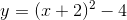Possible Answers: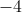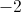Correct answer:Explanation:

To find the slope of a line tangent to a parabola at a specific point, find the derivative of the parabola's equation, then substitute the-coordinate of the specific point in the new equation.

In this case, it helps to expand the equation before taking the derivative: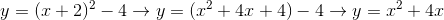Now take the derivative of the expanded equation: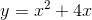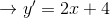Since the-intercept is the point where the-coordinate is, substituteinto the equation for.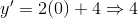### All High School Math Resources